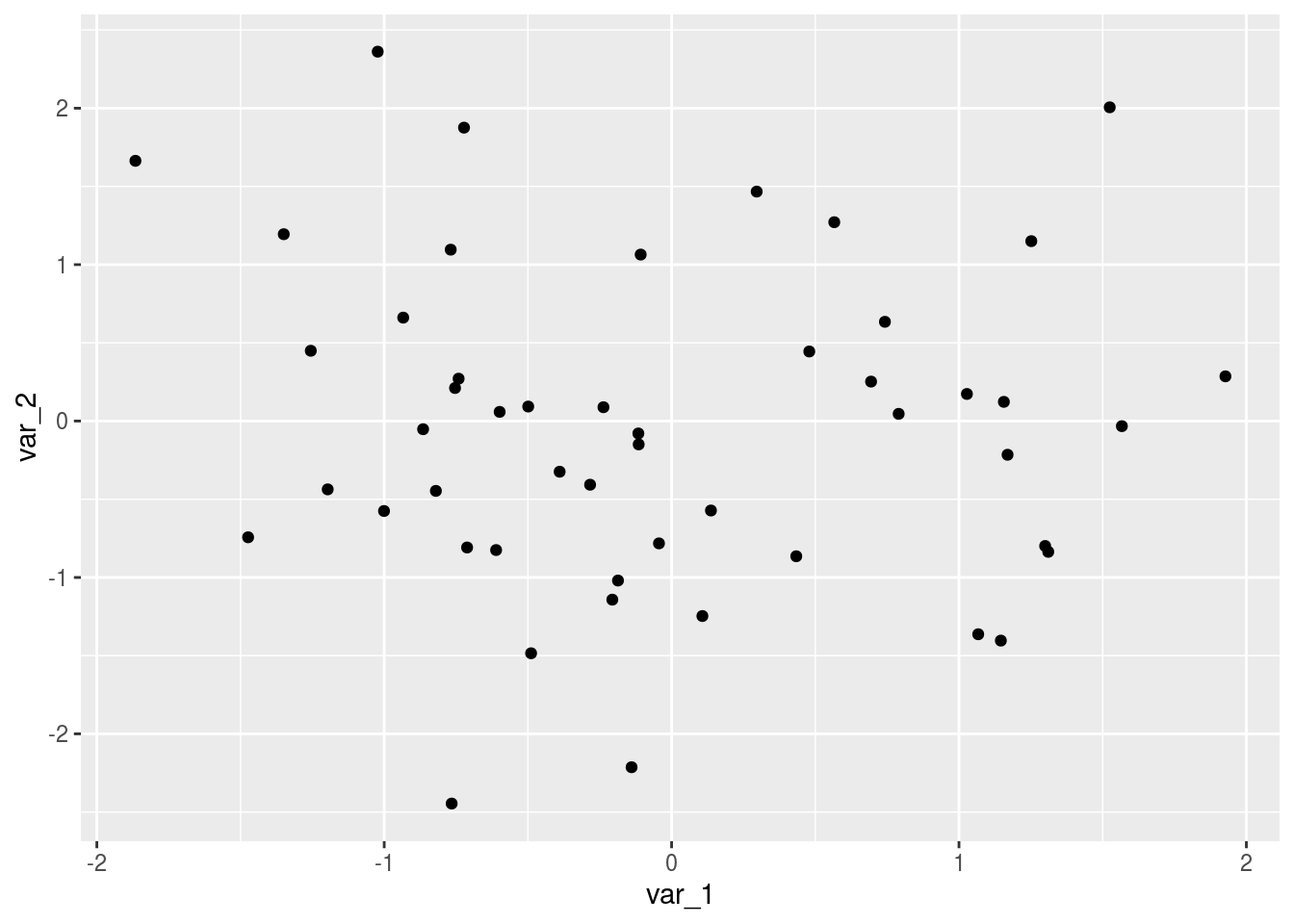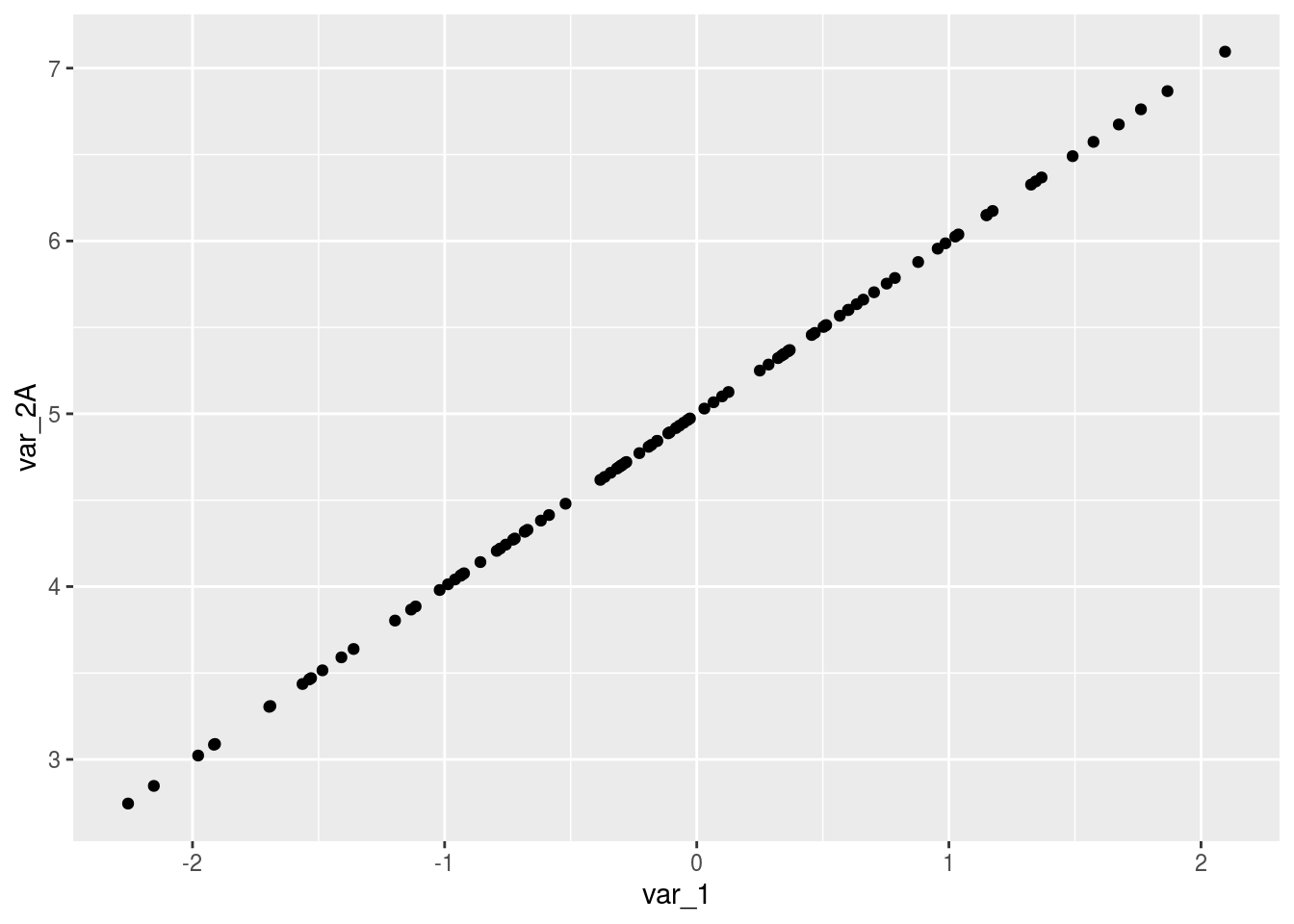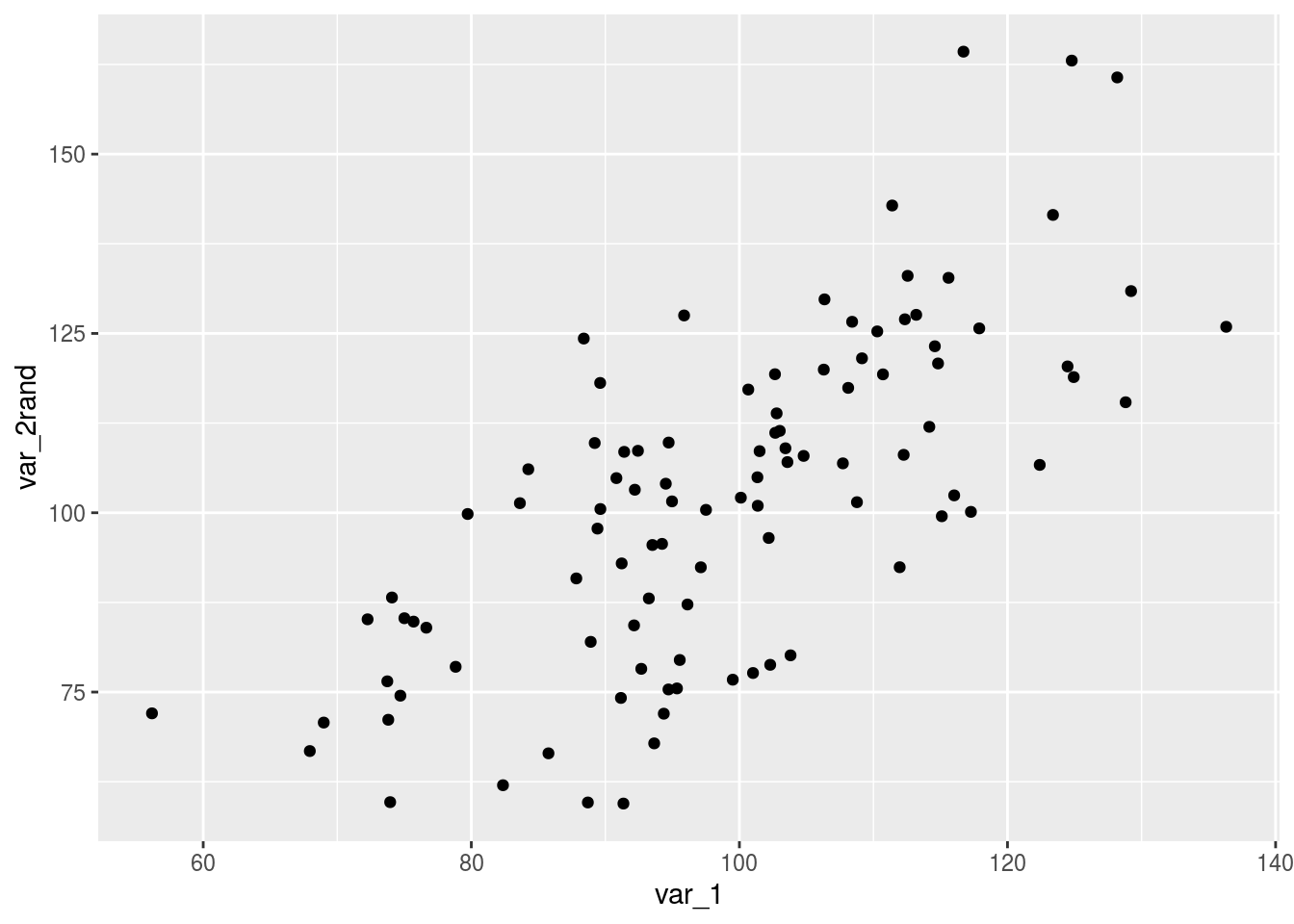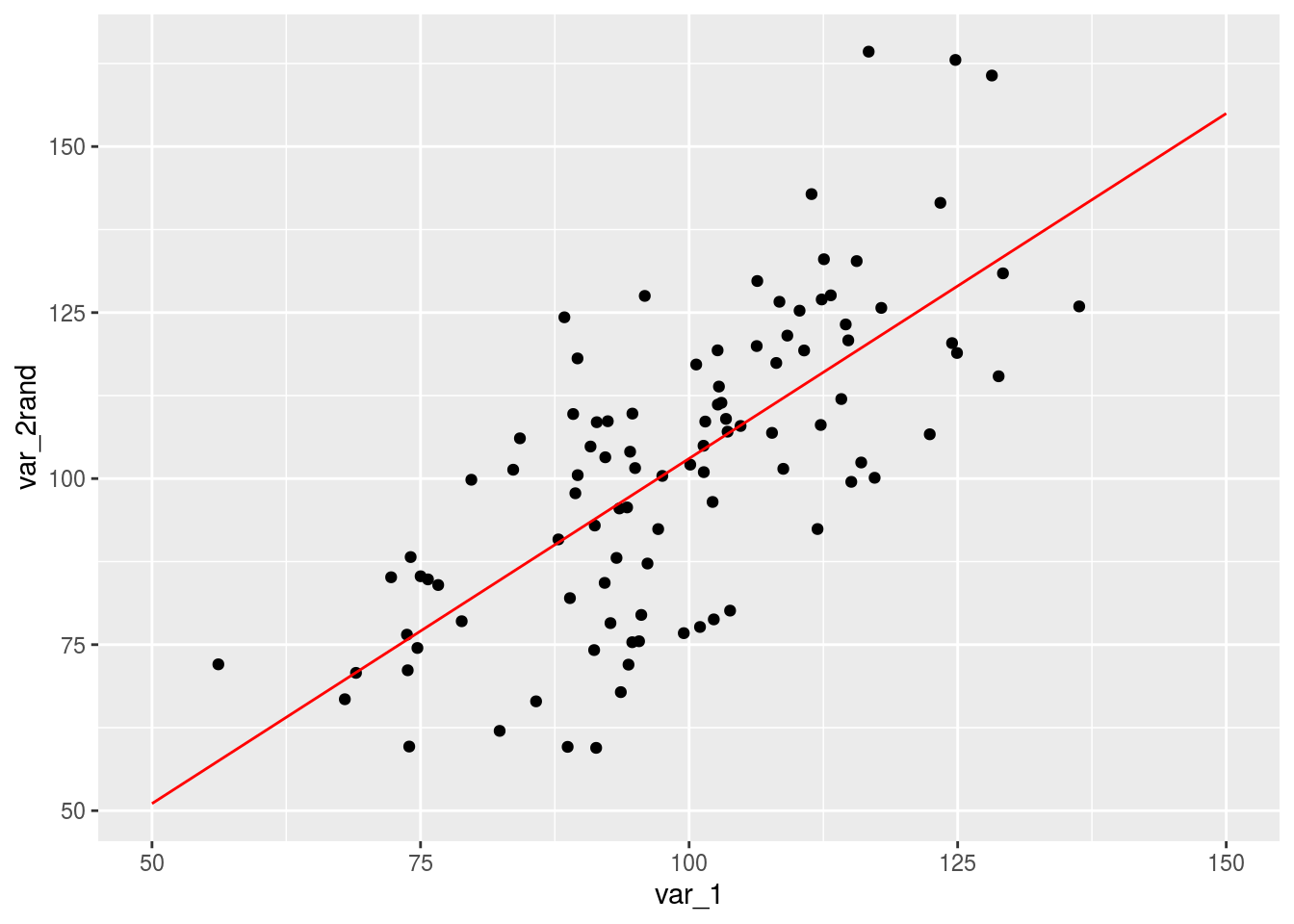In this lab, we’ll introduce two of the most important concepts in statistics: correlation and linear regression. Both of those concepts deal with the straifght line. Let’s see how we can compute the correlation between two variables and then look into linear regression.

# Correlation

Correlation is a measure of two things: magnitude of relationship between two variables, and the direction of this relationship. It ranges from -1 to +1, with 0 being the lowest correlation value possible. What do we mean when we say that two variables are correlated?

We’ll of course answer this question using simulations!

In this first simulaiton, we will create two variables, both are drawn from a normal distribution. Let’s also plot those two variables.

var_1 <- rnorm(50)
var_2 <- rnorm(50)
library(ggplot2)
ggplot(data.frame(var_1, var_2), aes(x=var_1,y=var_2)) +
geom_point()What do we see ? just scatter of random points. We don’t really expect those to have any relationship because each point is a random sample from a normal distribution. To make sure this is the case, let’s run a correlation test:

cor.test(var_1, var_2)
##
##  Pearson's product-moment correlation
##
## data:  var_1 and var_2
## t = -0.36969, df = 48, p-value = 0.7132
## alternative hypothesis: true correlation is not equal to 0
## 95 percent confidence interval:
##  -0.3267853  0.2284516
## sample estimates:
##         cor
## -0.05328437

which gives us a very low correlation (=0.1), with a high probability that the true correlation is actually zero.

Linear relationships mean that one variable is, to some degree, dependent on another variable. Dependence means that an offset (some value) is added or multipled to all numbers in the first list, making it perfectly dependent. To make two dependent variables, we’ll first make random numbers, but then we’ll see how we can create the second list of random numbers that are perfectly dependent on the first list by either addition or multiplication:

offset <- 5
N <- 100
var_1 <- rnorm(N)
var_2A <- var_1 + offset # just add 5 to each number: perfect relationship
var_2B <- var_1 * offset
var_2C <- var_1 * offset + offset

Now what do you think is the correlation between the two sets of numbers? And if we plot those relationships, what are you going to see?

cor(var_1, var_2A) 
##  1
cor(var_1, var_2B) 
##  1
cor(var_1, var_2C) 
##  1

Now let’s plot any of those

library(ggplot2)
ggplot(data.frame(var_1, var_2A), aes(x=var_1,y=var_2A)) +
geom_point()They are perfectly correlated. Meaning, if you give me any number from either list, I can give you the corresponding number from the other list. Well, that’s what we did by adding or multiplying by some amount.

Addition and multiplication are examples of linear transformations. Those transformations, however, only exist in an ideal mathematical world. Nature is filled with randomness – at least that’s what we are used to deal with. So let’s introduce some randomness into those transformnations. We introduce randomness by adding another set of random values to each point, which will either move it up or down by a small margin.

offset <- 5
N <- 100
var_1 <- rnorm(N, mean=100, sd=15)
var_2rand <- var_1 + offset + rnorm(N, sd=15)

We could have also multipled by that random vector (so each number will be scaled by that random amount). Now, let’s look at the plot:

library(ggplot2)
ggplot(data.frame(var_1, var_2rand), aes(x=var_1,y=var_2rand)) +
geom_point()And here we see a slightly more believable simulaiton of the relationship between two variables. We no longer have a perfect relationship, and when you give me any number on the x-axis, I can only give you a rough estimate of its corresponding y-value. Let’s see the correlation of those two:

cor(var_1, var_2rand)
##  0.7090511

You are probably thinking about drawing a line – but before we go there, let’s think about what is the graphical interpretation of the correlation between two variables, which will be relevant when we talk about liner regression. Please visit this site and play around with the simulations there: https://phet.colorado.edu/sims/html/least-squares-regression/latest/least-squares-regression_en.html

# Linear Regression

Now let’s talk about linear regression. In linear regression, we actually move one step ahead and describe the best-fit line with an equation – a very simple equation: y = slope * x + intercept. But why go the extra mile? Let’s assume that you studied verbal fluency across different age groups and you have a sample of 14 subjects with ages that range from 6 years old to 59 years old, and you already know their verbal fluency scores. You found a positive correlation between the two and you think you are done. You might know the true correlation between the two variable, but that does not mean you can predict the verbal fluency score using any value of age. More generally, we want to go from a purly descriptive number (like correlation) to a model that can describe the relationship between the two variables. This is the reason we talk about linear regression.

In the case of perfect linear relationship between two variables (i.e., correlation =1 or -1), we can simply calculate the slope by dividing the change in Y by change in X (or, rise by run). However, if the relationshio is imperfect, we’ll need a special formula that can find the best values for slope and intercept of the best-fitting line. Let’s see how we can do this in R with lm short of linear model.

linear_model <- lm(var_2rand ~ var_1)
linear_model
##
## Call:
## lm(formula = var_2rand ~ var_1)
##
## Coefficients:
## (Intercept)        var_1
##     -0.8838       1.0391

Here, you see that the value of the intercept is under the intercept column, and the value of the slope is under the name of the variable (var_1). If you want to make sure that it is indeed the case, we can make many points in the x-axis, and then use the straight line equations (with those parameters) to plot the y-axis. We should expect the line to be the best-fitting line.

x_points <- seq(50,150, 1)
y_points <- x_points * linear_model$coefficients + linear_model$coefficients
library(ggplot2)
ggplot(data.frame(var_1, var_2rand), aes(x=var_1,y=var_2rand)) +
geom_point() +
geom_line(data=data.frame(x_points, y_points), aes(x=x_points,y=y_points), color='red')Isn’t that beautiful? We simply created many points, as described by the straight line equation, and they indeed were along the line that best fit those points.

Linear regression, however, is not too far from correlation. If the standard deviations of the two variables are equal, then correlation will be the same as slope. Actually, slope is correlation, but scaled by the ratio of the standard deviation in y to the standard deviation in x. If you know the correlation between x and y, and the standard deviations, you can get the slope in a blink of an eye.

# this is the slope
(sd(var_2rand)/sd(var_1))*cor(var_1, var_2rand)
##  1.039083

# Inferential statistics

Of course, we are only doing those calculations on a sample to estimate something about a population (are you having a deja-vu?). The question now is: how to calculate those inferential estimates in R? Let’s be clear here: we want to estimate the true values of intercept and slope in the population based on a sample data. So, we’ll have two separate estimates, and with each estimate we have a confidence interval and an error. In R, you need not to worry because we already have those values calculated for us – just use summary:

summary(lm(var_2rand ~ var_1))
##
## Call:
## lm(formula = var_2rand ~ var_1)
##
## Residuals:
##     Min      1Q  Median      3Q     Max
## -34.578 -10.586   0.494  11.176  43.891
##
## Coefficients:
##             Estimate Std. Error t value Pr(>|t|)
## (Intercept)  -0.8838    10.4518  -0.085    0.933
## var_1         1.0391     0.1044   9.954   <2e-16 ***
## ---
## Signif. codes:  0 '***' 0.001 '**' 0.01 '*' 0.05 '.' 0.1 ' ' 1
##
## Residual standard error: 16.23 on 98 degrees of freedom
## Multiple R-squared:  0.5028, Adjusted R-squared:  0.4977
## F-statistic: 99.09 on 1 and 98 DF,  p-value: < 2.2e-16

And you see a full report of the inferential stuff. First, notice that we have a standard error of each estimate, and from that standard error we can calculate everything. In linear regression, the Null Hypothesis is that the coefficients associated with the variables is equal to zero. The alternate hypothesis is that the coefficients are not equal to zero. With this, you can interpret the t-value and the p-value.

Let’s actually derive those value by ourselves.

The residual standard error is the standard deviation by the square root of the degrees of freedom. Here, we no longer deal with the standard deviation of the mean, but rather the standard deviation from the fitted line.

# MSE = true Y -  predicted Y
predicted_y <- var_1 * linear_model$coefficients + linear_model$coefficients
SSE <- sum((var_2rand - predicted_y)^2) # SSE
q <- 2 # here, we are estimating 2 numbers: slope and intercept (we used to estimate only the mean and hence set this value to 1)
df <- N - q
MSE <- SSE/df
SEM <- sqrt(MSE)
SEM
##  16.23326

# Exercises

• Conduct a small power analysis to determine the minimum N needed to acheive a power of 80% using a correlation test (i.e.,cor.test(x,y)). To do this, you need to use random numbers from rnorm.
N<-10
variable_1 <- rnorm(N, mean=0, sd=1)
variable_2 <- rnorm(N, mean=0, sd=1)
corr_val <- cor.test(variable_1, variable_2)
p_value <- corr_val # this is the p-value
p_value
## \$p.value
##  0.1727854
• R^2 is called the coefficient of determination and it is usually used as a measure of the effect size. Conduct another power analysis that shows the numimum effect size needed to achieve 80% power using N = 20.